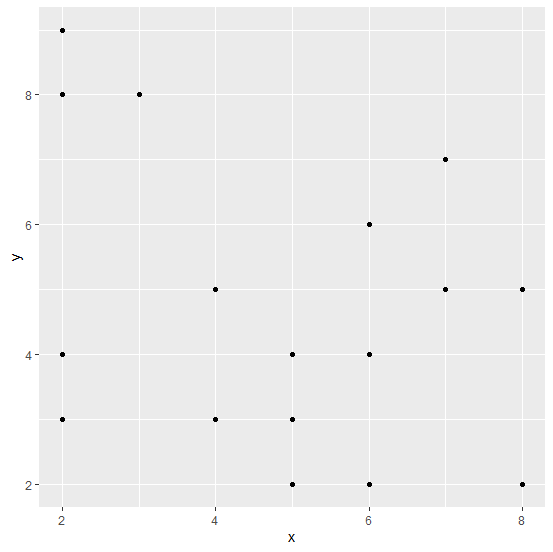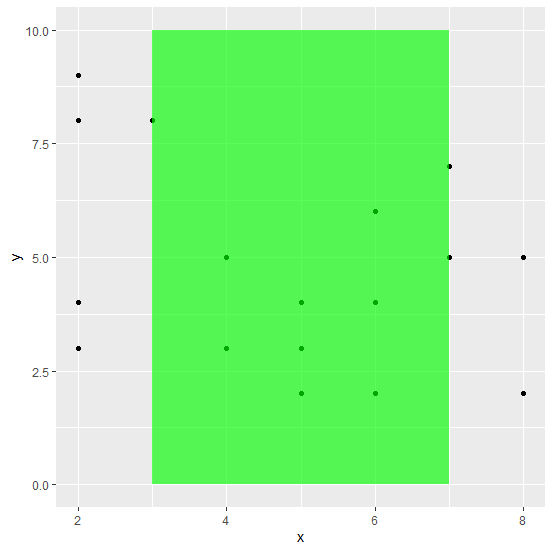# How to change the partial plot background using ggplot2 in R?

To change the partial plot background, we can create a rectangle using geom_rect function by defining both the axes values and alpha for transparency, the color will be changed by using fill argument. The value of alpha will completely hide the grey background and we can play with its value based on our need.

## Example

Live Demo

Consider the below data frame −

x<−rpois(20,5)
y<−rpois(20,5)
df<−data.frame(x,y)
df

## Output

x y
1 5 4
2 4 5
3 4 3
4 6 2
5 7 5
6 2 4
7 3 8
8 7 5
9 2 3
10 5 3
11 7 7
12 5 4
13 8 2
14 6 4
15 3 8
16 2 9
17 5 2
18 8 5
19 2 8
20 6 6

Loading ggplot2 package and creating a point chart between x and y −

## Example

library(ggplot2)
ggplot(df,aes(x,y))+geom_point()

## OutputCreating a rectangle inside the plot to change the background of the plot −

## Example

ggplot(df,aes(x,y))+geom_point()+geom_rect(aes(xmin=3,xmax=7,ymin=0,ymax=10),fill="green",alpha=0.05)

## Output